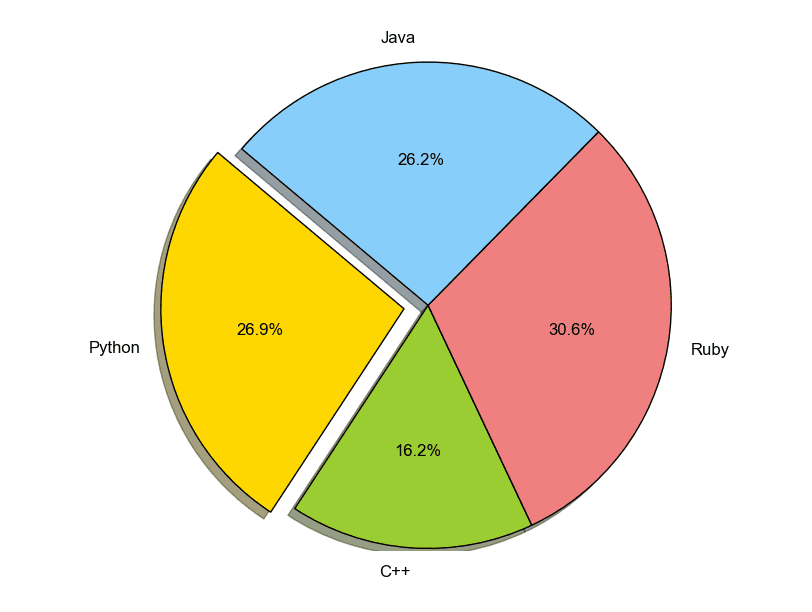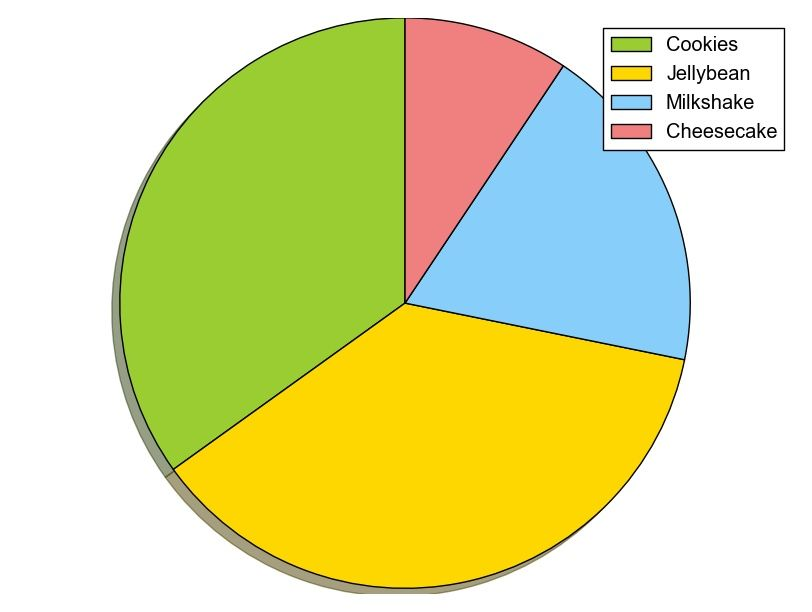# Matplotlib Pie chart

Python hosting: Host, run, and code Python in the cloud!

Matplotlib supports pie charts using the pie() function. You might like the Matplotlib gallery.

The matplotlib module can be used to create all kinds of plots and charts with Python. A pie chart is one of the charts it can create, but it is one of the many.

Related course: Data Visualization with Matplotlib and Python

## Matplotlib pie chart

First import plt from the matplotlib module with the line import matplotlib.pyplot as plt
Then you can use the method plt.pie() to create a plot.

The code below creates a pie chart:

The above code has the following output:pie chart python

You can define it’s sizes, which parts should explode (distance from center), which labels it should have and which colors it should have.

## Matplotlib pie chart legend

To add a legend use the plt.legend() function. This adds a legend on top of the plot.

It outputs this plot:python pie chart

While making the plot, don’t forget to call the method .show().